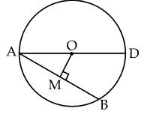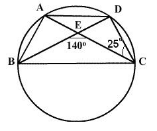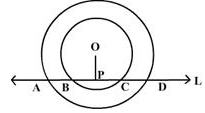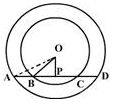Courses

# Test: Perpendicular From Centre To Chord

## 10 Questions MCQ Test Mathematics (Maths) Class 9 | Test: Perpendicular From Centre To Chord

Description
This mock test of Test: Perpendicular From Centre To Chord for Class 9 helps you for every Class 9 entrance exam. This contains 10 Multiple Choice Questions for Class 9 Test: Perpendicular From Centre To Chord (mcq) to study with solutions a complete question bank. The solved questions answers in this Test: Perpendicular From Centre To Chord quiz give you a good mix of easy questions and tough questions. Class 9 students definitely take this Test: Perpendicular From Centre To Chord exercise for a better result in the exam. You can find other Test: Perpendicular From Centre To Chord extra questions, long questions & short questions for Class 9 on EduRev as well by searching above.
QUESTION: 1

Solution:
QUESTION: 2

Solution:
QUESTION: 3

### The length of chord which is at a distance of 12 cm from centre of circle of radius 13 cm is:

Solution:
QUESTION: 4

AD is a diameter of a circle and AB is chord. If AB = 24 cm, AD = 30 cm, the distance of AB from the centre of the circle is :Solution:
QUESTION: 5

The perpendicular distance of a chord 8 cm long from the centre of a circle of radius 5 cm is

Solution:
QUESTION: 6

A, B, C and D are four points on a circle. AC and BD intersect at E such that angle BEC = 140° and angle ECD = 25°, then angle BAC isSolution:
QUESTION: 7

The radius of a circle which has a 6 cm long chord, 4 cm away from the centre of the circle is

Solution:
QUESTION: 8

Two concentric circles are intersected by a line L at A, B, C and D. ThenSolution:
QUESTION: 9

In the figure, AD is a straight line. OP is perpendicular to AD and O is the centre of both the circles. If AO = 34 cm, OB = 20 cm and OP = 16 cm, then the length of AD isSolution:
QUESTION: 10

Two circles of radii10 cm and 8 cm intersect and the length of the common chord is 12 cm. The distance between their centers is

Solution:

Center of circles= O and O'